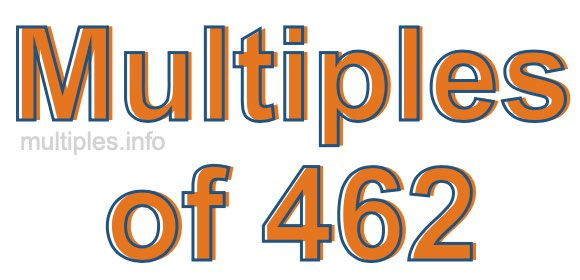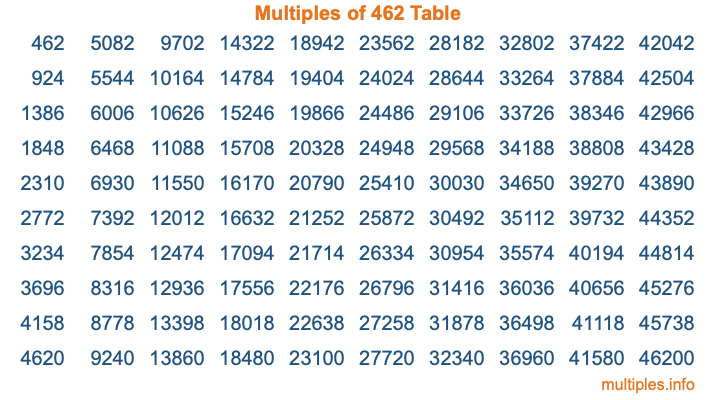Multiples of 462Welcome to the Multiples of 462 page. Here we will first teach you everything you will ever need to know about the multiples of 462, and then give you a study guide summary of everything we taught you to make sure you remember it all. Use this page to look up facts and learn information about the multiples of 462. This page will make you a multiples of four hundred sixty-two expert!

Definition of Multiples of 462
Multiples of 462 are all the numbers that when divided by 462 equal an integer. Each of the multiples of 462 are called a multiple. A multiple of 462 is created by multiplying 462 by an integer.

Therefore, to create a list of multiples of 462, you start with 1 multiplied by 462, then 2 multiplied by 462, then 3 multiplied by 462, and so on for as long as you want. Thus, the list of the first five multiples of 462 is 462, 924, 1386, 1848, and 2310. To see a larger list of multiples of 462, see the printable image of Multiples of 462 further down on this page. We also have a category where you can choose any nth multiple of 462.

Multiples of 462 Checker
The Multiples of 462 Checker below checks to see if any number of your choice is a multiple of 462. In other words, it checks to see if there is any number (integer) that when multiplied by 462 will equal your number. To do that, we divide your number by 462. If the the quotient is an integer, then your number is a multiple of 462.

Is  a multiple of 462?

Least Common Multiple of 462 and ...
A Least Common Multiple (LCM) is the lowest multiple that two or more numbers have in common. This is also called the smallest common multiple or lowest common multiple and is useful to know when you are adding our subtracting fractions. Enter one or more numbers below (462 is already entered) to find the LCM.

Check out our LCM Calculator if you need more details about the Least Common Multiple or if you need the LCM for different numbers for adding and subtraction fractions.

nth Multiple of 462
As we stated above, 462 is the first multiple of 462, 924 is the second multiple of 462, 1386 is the third multiple of 462, and so on. Enter a number below to find the nth multiple of 462.

th multiple of 462

Multiples of 462 vs Factors of 462
462 is a multiple of 462 and a factor of 462, but that is where the similarities end. All postive multiples of 462 are 462 or greater than 462. All positive factors of 462 are 462 or less than 462.

Below is the beginning list of multiples of 462 and the factors of 462 so you can compare:

Multiples of 462: 462, 924, 1386, 1848, 2310, etc.

Factors of 462: 1, 2, 3, 6, 7, 11, 14, 21, 22, 33, 42, 66, 77, 154, 231, 462

As you can see, the multiples of 462 are all the numbers that you can divide by 462 to get a whole number. The factors of 462, on the other hand, are all the whole numbers that you can multiply by another whole number to get 462.

It's also interesting to note that if a number (x) is a factor of 462, then 462 will also be a multiple of that number (x).

Multiples of 462 vs Divisors of 462
The divisors of 462 are all the integers that 462 can be divided by evenly. Below is a list of the divisors of 462.

Divisors of 462: 1, 2, 3, 6, 7, 11, 14, 21, 22, 33, 42, 66, 77, 154, 231, 462

The interesting thing to note here is that if you take any multiple of 462 and divide it by a divisor of 462, you will see that the quotient is an integer.

Multiples of 462 Table
Below is an image of the first 100 multiples of 462 in a table. The table is in chronological order, column by column. The first column has the first ten multiples of 462, the second column has the next ten multiples of 462, and so on.The Multiples of 462 Table is also referred to as the 462 Times Table or Times Table of 462. You are welcome to print out our table for your studies.

Negative Multiples of 462
Although not often discussed or needed in math, it is worth mentioning that you can make a list of negative multiples of 462 by multiplying 462 by -1, then by -2, then by -3, and so on, to get the following list of negative multiples of 462:

-462, -924, -1386, -1848, -2310, etc.

Multiples of 462 Summary
Below is a summary of important Multiples of 462 facts that we have discussed on this page. To retain the knowledge on this page, we recommend that you read through the summary and explain to yourself or a study partner why they hold true.

There are an infinite number of multiples of 462.

A multiple of 462 divided by 462 will equal a whole number.

462 divided by a factor of 462 equals a divisor of 462.

The nth multiple of 462 is n times 462.

The largest factor of 462 is equal to the first positive multiple of 462.

462 is a multiple of every factor of 462.

462 is a multiple of 462.

A multiple of 462 divided by a divisor of 462 equals an integer.

462 divided by a divisor of 462 equals a factor of 462.

Any integer times 462 will equal a multiple of 462.

Multiples of a Number
Here you can get the multiples of another number, all with the same attention to detail as we did for multiples of 462 on this page.

Multiples of
Multiples of 463
Did you find our page about multiples of four hundred sixty-two educational? Do you want more knowledge? Check out the multiples of the next number on our list!